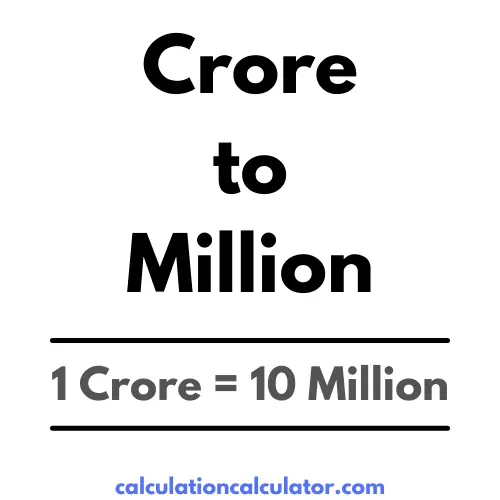# Crore to Million Conversion

## 1 Crore is equal to how many Million?

### 10 Million

##### Reference This Converter:Crore and Million both are the Number calculation unit. Compare values between unit Crore with other Number calculation units. You can also calculate other Number conversion units that are available on the select box, having on this same page.

Crore to Million conversion allows you to convert value between Crore to Million easily. Just enter the Crore value into the input box, the system will automatically calculate Million value. 1 Crore in Million? In mathematical terms, 1 Crore = 10 Million.

To conversion value between Crore to Million, just multiply the value by the conversion ratio. One Crore is equal to 10 Million, so use this simple formula to convert -

The value in Million is equal to the value of Crore multiplied by 10.

Million = Crore * 10;

For calculation, here's how to convert 10 Crore to Million using the formula above -

10 Crore = (10 * 10) = 100 Million
Crore Million Conversion
1 10 1 Crore = 10 Million
2 20 2 Crore = 20 Million
3 30 3 Crore = 30 Million
4 40 4 Crore = 40 Million
5 50 5 Crore = 50 Million
6 60 6 Crore = 60 Million
7 70 7 Crore = 70 Million
8 80 8 Crore = 80 Million
9 90 9 Crore = 90 Million
10 100 10 Crore = 100 Million
11 110 11 Crore = 110 Million
12 120 12 Crore = 120 Million
13 130 13 Crore = 130 Million
14 140 14 Crore = 140 Million
15 150 15 Crore = 150 Million
16 160 16 Crore = 160 Million
17 170 17 Crore = 170 Million
18 180 18 Crore = 180 Million
19 190 19 Crore = 190 Million
20 200 20 Crore = 200 Million
21 210 21 Crore = 210 Million
22 220 22 Crore = 220 Million
23 230 23 Crore = 230 Million
24 240 24 Crore = 240 Million
25 250 25 Crore = 250 Million
26 260 26 Crore = 260 Million
27 270 27 Crore = 270 Million
28 280 28 Crore = 280 Million
29 290 29 Crore = 290 Million
30 300 30 Crore = 300 Million
31 310 31 Crore = 310 Million
32 320 32 Crore = 320 Million
33 330 33 Crore = 330 Million
34 340 34 Crore = 340 Million
35 350 35 Crore = 350 Million
36 360 36 Crore = 360 Million
37 370 37 Crore = 370 Million
38 380 38 Crore = 380 Million
39 390 39 Crore = 390 Million
40 400 40 Crore = 400 Million
41 410 41 Crore = 410 Million
42 420 42 Crore = 420 Million
43 430 43 Crore = 430 Million
44 440 44 Crore = 440 Million
45 450 45 Crore = 450 Million
46 460 46 Crore = 460 Million
47 470 47 Crore = 470 Million
48 480 48 Crore = 480 Million
49 490 49 Crore = 490 Million
50 500 50 Crore = 500 Million
51 510 51 Crore = 510 Million
52 520 52 Crore = 520 Million
53 530 53 Crore = 530 Million
54 540 54 Crore = 540 Million
55 550 55 Crore = 550 Million
56 560 56 Crore = 560 Million
57 570 57 Crore = 570 Million
58 580 58 Crore = 580 Million
59 590 59 Crore = 590 Million
60 600 60 Crore = 600 Million
61 610 61 Crore = 610 Million
62 620 62 Crore = 620 Million
63 630 63 Crore = 630 Million
64 640 64 Crore = 640 Million
65 650 65 Crore = 650 Million
66 660 66 Crore = 660 Million
67 670 67 Crore = 670 Million
68 680 68 Crore = 680 Million
69 690 69 Crore = 690 Million
70 700 70 Crore = 700 Million
71 710 71 Crore = 710 Million
72 720 72 Crore = 720 Million
73 730 73 Crore = 730 Million
74 740 74 Crore = 740 Million
75 750 75 Crore = 750 Million
76 760 76 Crore = 760 Million
77 770 77 Crore = 770 Million
78 780 78 Crore = 780 Million
79 790 79 Crore = 790 Million
80 800 80 Crore = 800 Million
81 810 81 Crore = 810 Million
82 820 82 Crore = 820 Million
83 830 83 Crore = 830 Million
84 840 84 Crore = 840 Million
85 850 85 Crore = 850 Million
86 860 86 Crore = 860 Million
87 870 87 Crore = 870 Million
88 880 88 Crore = 880 Million
89 890 89 Crore = 890 Million
90 900 90 Crore = 900 Million
91 910 91 Crore = 910 Million
92 920 92 Crore = 920 Million
93 930 93 Crore = 930 Million
94 940 94 Crore = 940 Million
95 950 95 Crore = 950 Million
96 960 96 Crore = 960 Million
97 970 97 Crore = 970 Million
98 980 98 Crore = 980 Million
99 990 99 Crore = 990 Million
100 1000 100 Crore = 1000 Million
A crore is equal to 100 lakh or ten million in the Indian numbering system. It is written as 1,00,00,000 with the local 2,2,3 style of digit group separator.
Million is used for counting the number, and it is equal to one thousand thousand. It is commonly abbreviated in British English as m, M, MM, mm, or mn in financial contexts.
The value in Million is equal to the value of Crore multiplied by 10.
Million = Crore * 10;
1 Crore is equal to 10 Million.
1 Crore = 10 Million.